Next: 1D Slab heated at Up: EXAMPLES, TEMPERATURE FROM GF Previous: 1D infinite body with

## Semi-infinite body heated at a type 1 boundary.

Consider the temperature in semi-infinite body with a specified temperature applied to the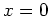boundary. The temperature satisfies the following equations: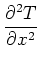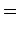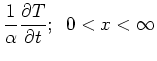(8)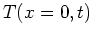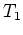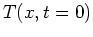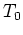The above problem has two non-homogeneous terms, however one may be eliminated to simplify the problem by normalizing the temperature. Let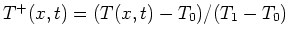. If you replace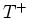into the above problem, the initial condition is zero and the boundary temperature is unity. Then this example is described by number X10B1T0. The temperature is given by the type 1 boundary term of the GF solution equation, as follows: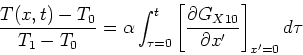(9)

Note that here the sign of the derivative has been chosen by the outward normal according to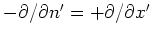. The GF is given by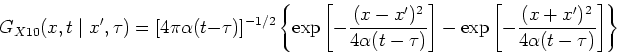(10)

The required derivative of the GF with respect to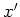evaluated at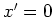is given by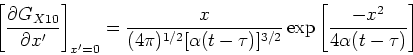(11)

Replace the above GF derivative into the GF solution equation to find the temperature; the integral may be evaluated as an error function.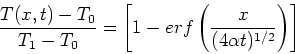(12)

or as the complementary error function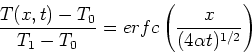(13)

Alternately, this problem could have been solved as an initial condition problem if the temperature were normalized as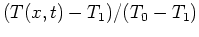.Next: 1D Slab heated at Up: EXAMPLES, TEMPERATURE FROM GF Previous: 1D infinite body with
2004-01-31